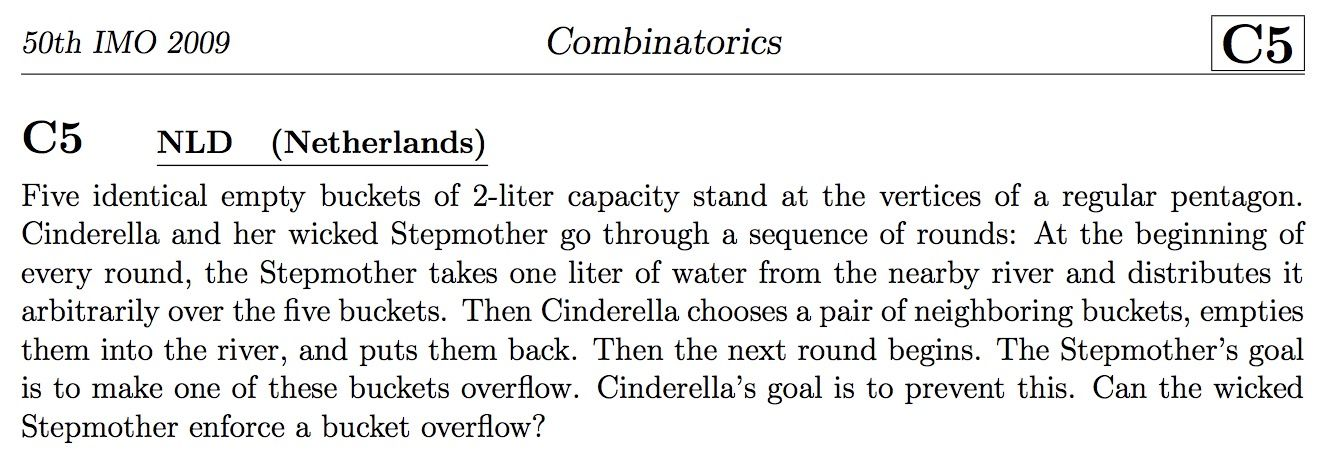시간 제한메모리 제한제출정답맞힌 사람정답 비율
2 초 512 MB51133.333%

## 문제Is it a notorious coincidence with this problem?

Cinderella and her wicked Stepmother are playing the game. Cinderella has $n$ non-negative integers $$a_1, a_2, \dots , a_n$$ at first. There are two parameters $A$ and $B$  for this game.

Cinderella and Stepmother take turns playing, starting with Cinderella. One each turn, Cinderella can replace the sequence $$a_1, a_2, \dots, a_n$$ by a new integer sequence $$a'_1, a'_2, \dots , a'_n$$ such that

•  $$\displaystyle a'_1 \ge a_1, \dots a'_n \ge a_n$$
• $$\displaystyle\sum_{i=1}^{n}{a'_i} \le \sum_{i=1}^{n}{a_i} + A$$

Then Stepmother can choose $B$ indices $i_1, i_2, \dots , i_B$, and set $a_{i_1}, a_{i_2}, \dots , a_{i_B}$ to 0.

The game continues forever. Let $M$ be the maximum value of $a_1, a_2, \dots , a_n$ for all the time. Cinderella wants to maximize $M$, and Stepmother wants to minimize $M$.

Determine the value of $M$ if both players play optimally.

## 입력

The first line contains an integer $T$ ($1 \le T \le 10^5$) indicating the number of test cases. For each test case:

The first line contains three integers $n, A, B$ ($1 \le B \le n \le 10^5, 0 \le A \le 10^{12}$).

The second line contains $n$ integers $a_1, a_2, \dots , a_n$ ($0 \le a_i \le 10^{12}$).

It is guaranteed that $$\sum{n} \le 5 \times 10^5$$.

## 출력

For each test case, output a line containing one integer: the answer.

## 예제 입력 1

4
3 5 1
1 2 3
5 5 1
0 2 1 0 3
5 100 5
1 2 3 4 5
8 3 1
5 1 2 2 0 2 5 1


## 예제 출력 1

11
14
105
9


## 힌트

A possible game process for the first test case:

{1, 2, 3} → {3, 4, 4} → {3, 4, 0} → {6, 6, 0} → {6, 0, 0} → {11, 0, 0}.